# Flow around the cylinder 2D single level

Navigate: ← Test Case Channel 2D

# Flow around the cylinder in a channel 2D

This example looks at the flow though a 2D channel with a cylinder placed into it and a mesh of multiple levels to better resolve the flow close to walls.

## Problem description

The Poiseuille flow is the fully developed laminar flow between two parallel plates induced by a constant pressure drop in a channel of length L. We place a cylinder into that channel as a disturbance and look at the flow around this cylinder. In general, the flow can be induced by any of the following way:

• Defining pressure at inlet and outlet of the channel.
• Defining velocity at inlet and pressure at outlet of the channel.
• Using pressure gradient i.e. pressure drop/length as a external force.

Here, the flow is induced by pressure boundary conditions at inlet (west) and outlet (east) boundaries.

The pressure drop along the channel per unit length is where,

• $\mathbf{U}_m$ - the maximum fluid velocity at the channel center axis,
• $\rho=1.0$ - the fluid density and
• $\nu=10^{-3} m^2/s$ - kinematic viscosity.

The Reynolds number is defined as where, $\bar{U}$ - the mean velocity. For the parabolic velocity profile, the mean velocity can be computed with $\mathbf{\bar U} = 2 \mathbf{U}_m /3$.

## Post-processing

Tracking relevant quantities gives us the following visualizations:

Lift coefficient of the cylinder over time: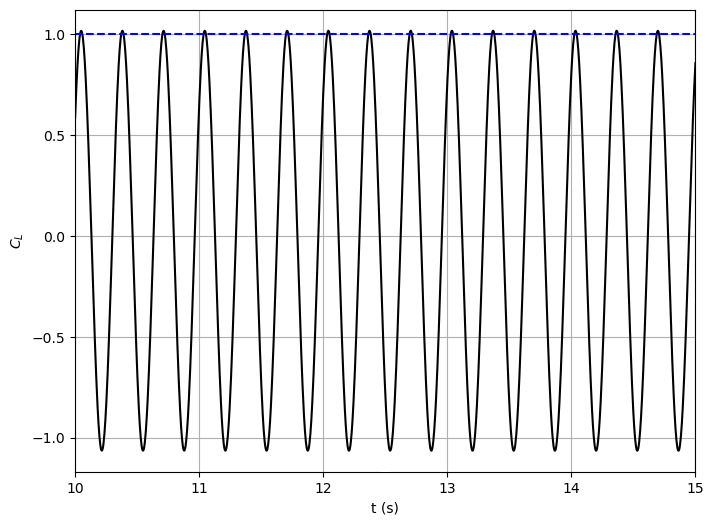Drag coefficient of the cylinder over time: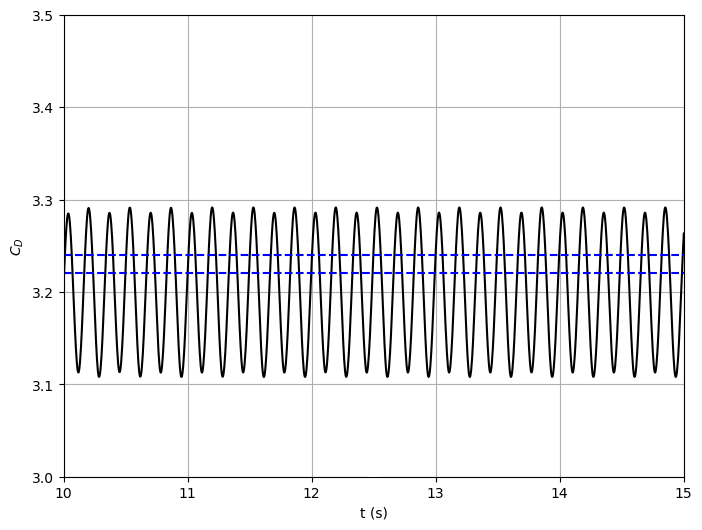Pressure along the length of the channel:Pressure coefficient over the cylinder surface:Pressure difference between stagnation in front of cylinder and back of the cylinder over time: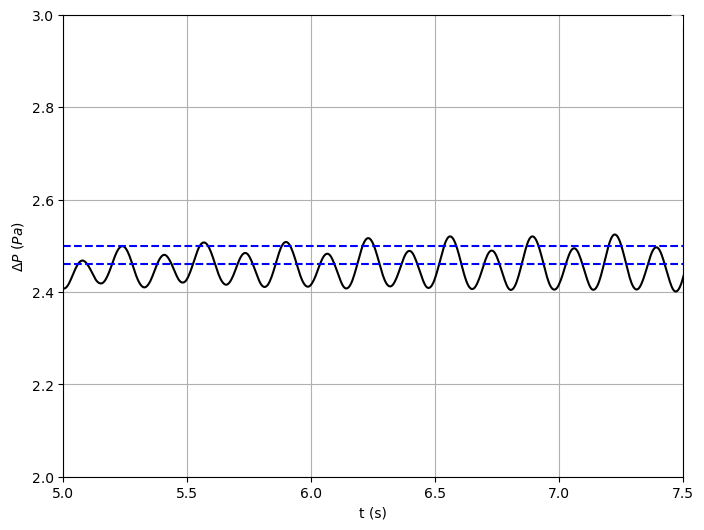The X-velocity component along the length of the channel: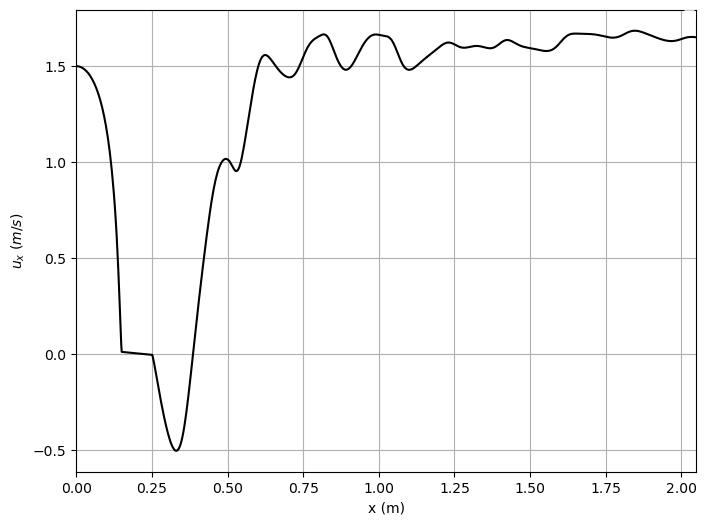The Y-velocity component along the length of the channel: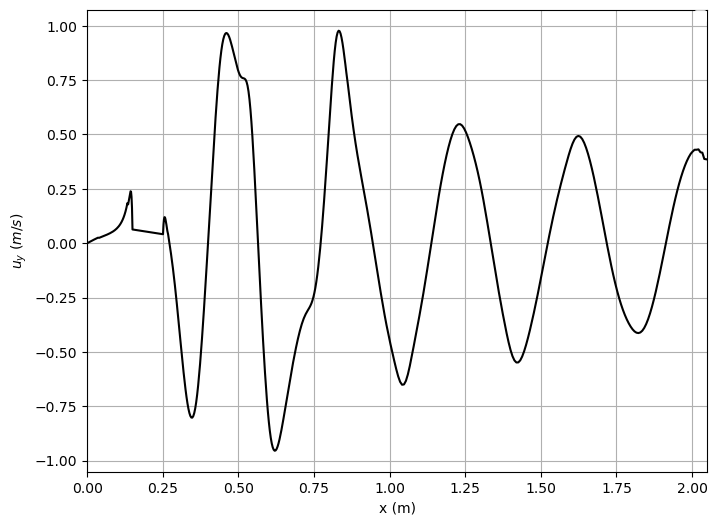A Fourier transform of the flow field:To create these plots, run python plot_track.py to create the plots. Before running the plot script, open 'plot_track.py' and update path to Gleaner script in 'glrPath'. Download Gleaner script using hg clone https://geb.inf.tu-dresden.de/hg/gleaner

Musubi was developed by University of Siegen